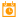# Date Time

 Block Group: Date Time Operations Icon:The Date Time block takes multiple inputs that represent a year, month, day, hour, minute, second, and millisecond and returns the sequential serial number that represents the date and time.

The Date Time block is useful when a date is supplied in a format that DGLux5 does not recognize, such as YYYYMMDD. You can use the Date Time block with other blocks, such as the Substring block, to convert the dates to a serial number that DGLux5 recognizes. See Scripting and Syntax.

For information on using dataflow blocks, see Dataflow.

## Input/Output Properties

The following properties of the Date Time block can take input and give output.

• year (number)
• month (number)
• day (number)
• hour (number)
• minute (number)
• second (number)
• millisecond (number)
• isUTC (boolean)

year specifies the year. Can include one to four digits.

month specifies the month of the year, from 1 to 12 (January to December). Can be a positive or negative integer.

day specifies the day of the month, from 1 to 31. Can be a positive or negative integer.

hour specifies the hour of the day, from 0 to 23. Can be a positive or negative integer.

minute specifies the minute of the hour, from 0 to 59. Can be a positive or negative integer.

second specifies the second of the minute, from 0 to 59. Can be a positive or negative integer.

millisecond specifies the millisecond of the second, from 0 to 999. Can be a positive or negative integer.

isUTC controls whether to use UTC. By default, this property is FALSE and DGLux5 uses your computer’s time zone.

## Output Property

The following property of the Date Time block can give output but cannot take input.

• output (number)

output returns the sequential serial number that represents a particular date and time.

## Special Cases

The following are special cases for the Date Time block:

• Integers greater than the maximum add to the date or time, while integers less than 1 subtract from the date or time. For example, with year equal to 2016 and month equal to 14, the output represents February 2017.
• All null values are treated as zero (0).

## Examples

The following image shows a typical example of the Date Time block. In this example, a serial number is created from the input properties.

The following image shows two special cases of the Date Time block. In the leftmost Date Time block, a value of 16 for the month causes the result to be the fourth month of the following year. In the rightmost Date Time block, a value of –12 for the hour causes the result to be noon of the previous day.